# Meta-analytic functional decoding

Functional decoding performed with meta-analytic data, refers to methods which attempt to predict mental states from neuroimaging data using a large-scale meta-analytic database (Smith et al., 2009). Such analyses may also be referred to as “informal reverse inference” (Poldrack, 2011), “functional characterization analysis” (Bzdok, Laird, et al., 2013; Cieslik et al., 2013; Rottschy et al., 2013), “open-ended decoding” (Rubin et al., 2017), or simply “functional decoding” (Amft et al., 2015; Bzdok, Langner, et al., 2013; Nickl-Jockschat et al., 2015 ). While the terminology is far from standardized, we will refer to this method as meta-analytic functional decoding in order to distinguish it from alternative methods like multivariate decoding and model-based decoding (Poldrack, 2011). Meta-analytic functional decoding is often used in conjunction with MACM, meta-analytic clustering, meta-analytic parcellation, and meta-ICA, in order to characterize resulting brain regions, clusters, or components. Meta-analytic functional decoding models have also been extended for the purpose of meta-analytic functional encoding, wherein text is used to generate statistical images (Dockès et al., 2018; Nunes, 2018; Rubin et al., 2017).

Four common approaches are correlation-based decoding, dot-product decoding, weight-sum decoding, and chi-square decoding. We will first discuss continuous decoding methods (i.e., correlation and dot-product), followed by discrete decoding methods (weight-sum and chi-square).

## Continuous decoding

When decoding unthresholded statistical maps, the most common approaches are to simply correlate the input map with maps from the database, or to compute the dot product between the two maps. In Neurosynth, meta-analyses are performed for each label (i.e., term or topic) in the database and then the input image is correlated with the resulting unthresholded statistical map from each meta-analysis. Performing statistical inference on the resulting correlations is not straightforward, however, as voxels display strong spatial correlations, and the true degrees of freedom are consequently unknown (and likely far smaller than the nominal number of voxels). In order to interpret the results of this decoding approach, users typically select some arbitrary number of top correlation coefficients ahead of time, and use the associated labels to describe the input map. However, such results should be interpreted with great caution.

This approach can also be applied to an image-based database like NeuroVault, either by correlating input data with meta-analyzed statistical maps, or by deriving distributions of correlation coefficients by grouping statistical maps in the database according to label. Using these distributions, it is possible to statistically compare labels in order to assess label significance. NiMARE includes methods for both correlation-based decoding and correlation distribution-based decoding, although the correlation-based decoding is better established and should be preferred over the correlation distribution-based decoding.

### Correlation-based decoding

CorrelationDecoder

The correlation-based decoding is implemented in NiMARE’s CorrelationDecoder class object.

from nimare.decode.continuous import CorrelationDecoder
from nimare.meta.cbma import mkda

decoder = CorrelationDecoder(
frequency_threshold=0.001,
meta_estimator=mkda.MKDAChi2,
target_image='z_desc-specificity',
)
decoder.fit(ns_dset)
decoding_results = decoder.transform('pain_map.nii.gz')


### Correlation distribution-based decoding

CorrelationDistributionDecoder

The distribution-based decoding is implemented in NiMARE’s CorrelationDistributionDecoder class object.

### The GCLDA approach

gclda_decode_map()

Recently, it was introduced a new decoding framework based on Generalized Correspondence LDA (GC-LDA, (Rubin et al., 2017)), an extension to the LDA model. This method, in addition to the two probability distributions from LDA (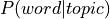and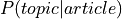), produces an additional probability distribution: the probability of a voxel given topic: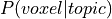, which we use to calculate the word weight associated with an input image.

The default implementation of the GC-LDA decoding in NiMARE, made use of a dot product continuous decoding approach: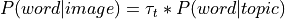where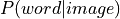is the vector of term/word weight associated with an input image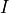(e.g., unthresholded statistical maps), and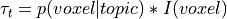is the topic weight vector,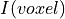is a vector with z-score value for each masked voxel of the input image.gives the most likely word from the top associated topics for a given unthresholded statistical map of interest.

To run th GC-LDA decoding approach, you need to train a GC-LDA model (see GCLDA topic modeling).

## Discrete decoding

Decoding regions of interest requires a different approach than decoding unthresholded statistical maps. One simple approach, used by GC-LDA, simply sums the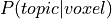distribution across all voxels in the ROI in order to produce a value associated with each topic for the ROI. These weight sum values are arbitrarily scaled and cannot be compared across ROIs.

One method which relies on correlations, much like the continuous correlation decoder, is the ROI association decoding method, originally implemented in the Neurosynth Python library. In this method, each study with coordinates in the dataset is convolved with a kernel transformer to produce a modeled activation map. The resulting modeled activation maps are then masked with a region of interest (i.e., the target of the decoding), and the values are averaged within the ROI. These averaged modeled activation values are then correlated with the term weights for all labels in the dataset. This decoding method produces a single correlation coefficient for each of the dataset’s labels.

A more theoretically driven approach to ROI decoding is to use chi-square-based methods. The two methods which use chi-squared tests are the BrainMap decoding method and an adaptation of Neurosynth’s meta-analysis method.

In both chi-square-based methods, studies are first selected from a coordinate-based database according to some criterion. For example, if decoding a region of interest, users might select studies reporting at least one coordinate within 5 mm of the ROI. Metadata (such as ontological labels) for this subset of studies are then compared to those of the remaining, unselected portion of the database in a confusion matrix. For each label in the ontology, studies are divided into four groups: selected and label-positive (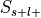), selected and label-negative (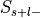), unselected and label-positive (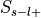), and unselected and label-negative (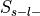). Each method then compares these groups in order to evaluate both consistency and specificity of the relationship between the selection criteria and each label, which are evaluated in terms of both statistical significance and effect size.

### The BrainMap approach

The BrainMap discrete decoding method compares the distributions of studies with each label within the sample against those in a larger database while accounting for the number of foci from each study. Broadly speaking, this method assumes that the selection criterion is associated with one peak per study, which means that it is likely only appropriate for selection criteria based around foci, such as regions of interest. One common analysis, meta-analytic clustering, involves dividing studies within a database into meta-analytic groupings based on the spatial similarity of their modeled activation maps (i.e., study-wise pseudo-statistical maps produced by convolving coordinates with a kernel). The resulting sets of studies are often functionally decoded in order to build a functional profile associated with each meta-analytic grouping. While these groupings are defined as subsets of the database, they are not selected based on the location of an individual peak, and so weighting based on the number of foci would be inappropriate.

This decoding method produces four outputs for each label. First, the distribution of studies in the sample with the label are compared to the distributions of other labels within the sample. This consistency analysis produces both a measure of statistical significance (i.e., a p-value) and a measure of effect size (i.e., the likelihood of being selected given the presence of the label). Next, the studies in the sample are compared to the studies in the rest of the database. This specificity analysis produces a p-value and an effect size measure of the posterior probability of having the label given selection into the sample. A detailed algorithm description is presented below.

The BrainMap method for discrete functional decoding performs both forward and reverse inference using an annotated coordinate-based database and a target sample of studies within that database. Unlike the Neurosynth approach, the BrainMap approach incorporates information about the number of foci associated with each study in the database.

1. Select studies in the database according to some criterion (e.g., having at least one peak in an ROI).

2. For each label, studies in the database can now be divided into four groups.

• Label-positive and selected –>• Label-negative and selected –>• Label-positive and unselected –>• Label-negative and unselected –>3. Additionally, the number of foci associated with each of these groups is extracted.

• Number of foci from studies with label,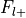• Number of foci from studies without label,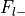• Total number of foci in the database,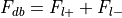4. Compute the number of times any label is used in the database,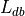(e.g., if every experiment in the database uses two labels, then this number is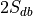, where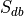is the total number of experiments in the database).

5. Compute the probability of being selected,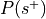.

•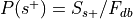, where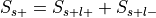6. For each label, compute the probability of having the label,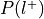.

•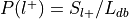, where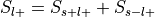7. For each label, compute the probability of being selected given presence of the label,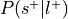.

• Can be re-interpreted as the probability of activating the ROI given a mental state.

•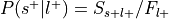8. Convertinto the forward inference likelihood,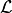.

•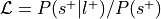9. Compute the probability of the label given selection,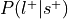.

• Can be re-interpreted as probability of a mental state given activation of the ROI.

•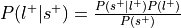• This is the reverse inference posterior probability.

10. Perform a binomial test to determine if the rate at which studies are selected from the set of studies with the label is significantly different from the base probability of studies being selected across the whole database.

• The number of successes is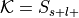, the number of trials is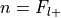, and the hypothesized probability of success is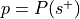• If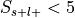, override the p-value from this test with 1, essentially ignoring this label in the analysis.

• Convert p-value to unsigned z-value.

11. Perform a two-way chi-square test to determine if presence of the label and selection are independent.

• If, override the p-value from this test with 1, essentially ignoring this label in the analysis.

• Convert p-value to unsigned z-value.

from nimare.decode.discrete import BrainMapDecoder

decoder = BrainMapDecoder(
frequency_threshold=0.001,
u=0.05,
correction='fdr_bh',
)
decoder.fit(ns_dset)
decoding_results = decoder.transform(amygdala_ids)


### The Neurosynth approach

The implementation of the MKDA Chi-squared meta-analysis method used by Neurosynth is quite similar to BrainMap’s method for decoding, if applied to annotations instead of modeled activation values. This method compares the distributions of studies with each label within the sample against those in a larger database, but, unlike the BrainMap method, does not take foci into account. For this reason, the Neurosynth method would likely be more appropriate for selection criteria not based on regions of interest (e.g., for characterizing meta-analytic groupings from a meta-analytic clustering analysis). However, the Neurosynth method requires user-provided information that BrainMap does not. Namely, in order to estimate probabilities for the consistency and specificity analyses with Bayes’ Theorem, the Neurosynth method requires a prior probability of a given label. Typically, a value of 0.5 is used (i.e., the estimated probability that an individual is undergoing a given mental process described by a label, barring any evidence from neuroimaging data, is predicted to be 50%). This is, admittedly, a poor prediction, which means that probabilities estimated based on this prior are not likely to be accurate, though they may still serve as useful estimates of effect size for the analysis.

Like the BrainMap method, this method produces four outputs for each label. For the consistency analysis, this method produces both a p-value and a conditional probability of selection given the presence of the label and the prior probability of having the label. For the specificity analysis, the Neurosynth method produces both a p-value and a posterior probability of presence of the label given selection and the prior probability of having the label. A detailed algorithm description is presented below.

The Neurosynth method for discrete functional decoding performs both forward and reverse inference using an annotated coordinate-based database and a target sample of studies within that database. Unlike the BrainMap approach, the Neurosynth approach uses an a priori value as the prior probability of any given experiment including a given label.

1. Select studies in the database according to some criterion (e.g., having at least one peak in an ROI).

2. For each label, studies in the database can now be divided into four groups:

• Label-positive and selected –>• Label-negative and selected –>• Label-positive and unselected –>• Label-negative and unselected –>3. Set a prior probability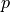of a given mental state occurring in the real world.

• Neurosynth uses 0.5 as the default.

4. Compute:

• Probability of being selected,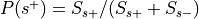, whereand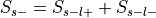5. For each label, compute:

•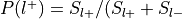, whereand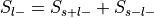6. Compute:

•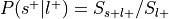7. Compute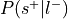:

•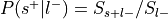• Only used to determine sign of reverse inference z-value.

8. Compute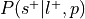, where is the prior probability of a label:

• This is the forward inference posterior probability. Probability of selection given label and given prior probability of label,.

•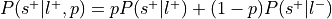9. Compute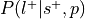:

• This is the reverse inference posterior probability. Probability of label given selection and given the prior probability of label.

•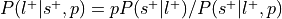10. Perform a one-way chi-square test to determine if the rate at which studies are selected for a given label is significantly different from the average rate at which studies are selected across labels.

• Convert p-value to signed z-value using whether the number of studies selected for the label is greater than or less than the mean number of studies selected across labels to determine the sign.

11. Perform a two-way chi-square test to determine if presence of the label and selection are independent.

• Convert p-value to signed z-value usingto determine sign.

from nimare.decode.discrete import NeurosynthDecoder

decoder = NeurosynthDecoder(
frequency_threshold=0.001,
u=0.05,
correction='fdr_bh',
)
decoder.fit(ns_dset)
decoding_results = decoder.transform(amygdala_ids)


### The Neurosynth ROI association approach

ROIAssociationDecoder

Neurosynth’s ROI association approach is quite simple, but it has been used in at least one publication, Margulies et al. (2016).

This approach uses the following steps to calculate label-wise correlation values:

1. Specify a region of interest (ROI) image in the same space as the Dataset.

2. Generate modeled activation (MA) maps for all studies in Dataset with coordinates.

3. Average the MA values within the ROI to get a study-wise MA regressor.

4. Correlate the MA regressor with study-wise annotation values (e.g., tf-idf values).

from nimare.decode.discrete import ROIAssociationDecoder

decoder = ROIAssociationDecoder(
"data/amygdala.nii.gz",
u=0.05,
correction="fdr_bh",
)
decoder.fit(ns_dset)
decoding_results = decoder.transform()


### The GC-LDA approach

gclda_decode_roi()

The GC-LDA approach sumsweights within the region of interest to produce topic-wise weights.

Example: Decode an ROI image

TBD